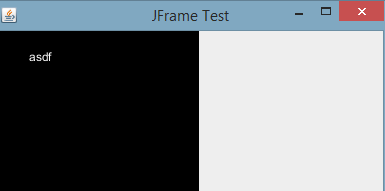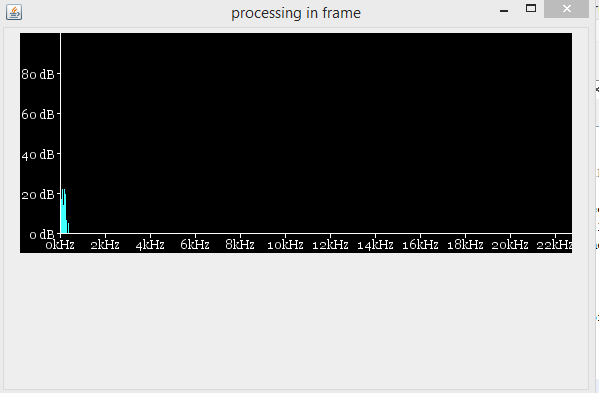#### Howdy, Stranger!

We are about to switch to a new forum software. Until then we have removed the registration on this forum.

# how can I run a processing sketch in a JPanel in Java.

edited April 2017

I have a JPanel where I want to run a processing sketch which performs spectrum analysis. I have tried psurface but it is giving me errors. Any Idea how to do it? Hope I will get answers this time. Thanks in advance

Tagged:

• AFAIK it is not possible to do this with Processing V3.

• In theory it might be possible to do with Processing 2.2.1

EDIT You would have to create a class that extends JPanel which has a field/attribute of type PApplet.

• edited April 2017

So I have to go for processing 2? Any link or material on how to do it? @quark

• I have no link or material on how to do it, my only experience comes from creating multiple windows for G4P.

I assume that you are using an IDE other than Processing e.g. Eclipse or Netbeans. What are you using?

There are some things you would need to consider

2) Create a new class (e.g. `MyPapplet`) that extends PApplet so you can override the normal Processing methods e.g. `setup()`, `draw()` etc.

3) Use a BorderLayout for the JPanel and add `papplet` to the CENTER to allow for resizing. If that causes problems use a FlowLayout.

That is how I would start. I can't promise that it will work so I suggest you create a new simple project to try these ideas out and then apply any solution to the spectrum analysis sketch.

WARNING
I did say

In theory it might be possible ...

so what I suggest here might not work, but whatever happens it will be very challenging.

• edited April 2017

Here is my code in java where I took a frame & inside a frame I took a psurface..And within that surface I tried to run a processing sketch to perform spectrum analysis. But its giving me errors.Like these:

``````**The sketch path is not set.
==== JavaSound Minim Error ====
==== Error invoking createInput on the file loader object: null

at ddf.minim.javasound.JSMinim.getAudioRecordingStream(Unknown Source)
at processingtest.ProcessingTest.setup(ProcessingTest.java:51)
at processing.core.PApplet.handleDraw(PApplet.java:2393)
at processing.awt.PSurfaceAWT\$12.callDraw(PSurfaceAWT.java:1540)
``````

If these codes can help..@GoToLoop @quark I would like to mention that psurface is working in jframe. Like the image below. But mine is not working.

TIA

```
package processingtest;

/**
*
* @author mezbahuddin
*/
import ddf.minim.AudioInput;
import ddf.minim.AudioPlayer;
import ddf.minim.Minim;
import ddf.minim.analysis.FFT;
import javax.swing.JFrame;

import processing.awt.PSurfaceAWT.SmoothCanvas;
import processing.core.PApplet;
import processing.core.PSurface;

public class ProcessingTest extends PApplet{

Minim minim;
AudioInput in;
FFT fft;
AudioPlayer ap;
// how many individual peak bands we have (dep. binsperband)
float gain = 0; // in dB
float dB_scale = (float) 2.0;  // pixels per dB
int buffer_size = 1024;  // also sets FFT size (frequency resolution)
float sample_rate = 44100;

int spectrum_height = 200; // determines range of dB shown
int legend_height = 20;
int spectrum_width = 512; // determines how much of spectrum we see
int legend_width = 40;
int freqOffset = 0;
public void settings() {
size(552, 220, "processing.opengl.PGraphics2D");
}
public void setup()
{
//size(552, 220, P2D);
//textMode(SCREEN);
textFont(createFont("Georgia", 12));

minim = new Minim(this);

// create an FFT object that has a time-domain buffer
// the same size as line-in's sample buffer
fft = new FFT(ap.bufferSize(), ap.sampleRate());

// Tapered window important for log-domain display
fft.window(FFT.HAMMING);

//println("buffer:"+in.bufferSize());
//println("sampleRate:"+in.sampleRate());
//println("peaksize:"+peaksize);
}
public void draw()
{
// clear window
background(0);

// perform a forward FFT on the samples in input buffer
fft.forward(ap.mix);
ap.play();

// now draw current spectrum in brighter blue
stroke(64,255,255);
noFill();
for(int i = 0; i < fft.specSize(); i++)  {

// draw the line for frequency band i using dB scale
float val = dB_scale*(20*((float)Math.log10(fft.getBand(i))) + gain);

if (fft.getBand(i) == 0) {   val = -200;   }  // avoid log(0)

int y = spectrum_height - Math.round(val);

if (y > spectrum_height) { y = spectrum_height; }

line(legend_width+i+freqOffset, spectrum_height, legend_width+i+freqOffset, y);

}
// frequency axis
fill(255);

stroke(255);

int y = spectrum_height;

line(legend_width,y,legend_width+spectrum_width,y); // horizontal line

// x,y address of text is immediately to the left of the middle of the letters
textAlign(CENTER,TOP);

int spFreq=0; //for spacing

for (float freq = (float) 0.0; freq < ap.sampleRate(); freq += 2000.0) {
int x = legend_width+spFreq+freqOffset; // which bin holds this frequency
//println(freq+"->"+fft.freqToIndex(freq));
line(x,y,x,y+4); // tick mark
text(Math.round(freq/1000) +"kHz", x, y+5); // add text label
spFreq+=45;
}

// DBlevel axis
int x = legend_width;

line(x,0,x,spectrum_height); // vertictal line

textAlign(RIGHT,CENTER);

for (float level = (float) -100.0; level < 100.0; level += 20.0) {
y = spectrum_height - (int)(dB_scale * (level+gain));

line(x,y,x-3,y);

text((int)level+" dB",x-5,y);
}

}
public static void main(String... args){

JFrame frame = new JFrame("JFrame Test");
frame.setDefaultCloseOperation(JFrame.EXIT_ON_CLOSE);

ProcessingTest pt = new ProcessingTest();

//get the PSurface from the sketch
PSurface ps = pt.initSurface();

//initialize the PSurface
ps.setSize(200, 200);

//get the SmoothCanvas that holds the PSurface
SmoothCanvas smoothCanvas = (SmoothCanvas)ps.getNative();

//SmoothCanvas can be used as a Component

frame.setSize(400, 200);
frame.setVisible(true);

}
}

```• In the code below I managed to get the sketch inside a JPanel but I also got the following error messages.

I always get lines 1-13 which I believe to be an Osx problem because Processing is trying to set the dock icon.

Notice line 13 - the sketch path hasn't been set :(

Once I added the Minim code I got the rest of the message.

There appears to be two problems line 16 and line 19 both caused by Minim and I suspect both caused by the fact the sketch path has not been set. Minim like most contributed libraries assume that the sketchPath is set and will attempt to use it.

I don't know what the solution is but if you find one please post it here.

``````java.lang.reflect.InvocationTargetException
at sun.reflect.NativeMethodAccessorImpl.invoke0(Native Method)
at sun.reflect.NativeMethodAccessorImpl.invoke(NativeMethodAccessorImpl.java:62)
at sun.reflect.DelegatingMethodAccessorImpl.invoke(DelegatingMethodAccessorImpl.java:43)
at java.lang.reflect.Method.invoke(Method.java:497)
at processing.awt.PSurfaceAWT.setProcessingIcon(PSurfaceAWT.java:654)
at processing.awt.PSurfaceAWT.initFrame(PSurfaceAWT.java:473)
at processing.core.PApplet.initSurface(PApplet.java:10636)
at ProcessingTest.main(ProcessingTest.java:61)
Caused by: java.lang.NullPointerException
at processing.core.ThinkDifferent.setIconImage(ThinkDifferent.java:88)
... 8 more
The sketch path is not set.
file:/Users/peter/git/quarks-stuff/My%20Processing%20Stuff/bin/data/Hall%20of%20the%20Mountain%20King.mp3
==== JavaSound Minim Error ====
==== java.lang.reflect.InvocationTargetException

=== Minim Error ===
=== Couldn't load the file file:/Users/peter/git/quarks-stuff/My%20Processing%20Stuff/bin/data/Hall%20of%20the%20Mountain%20King.mp3
``````

The Sketch

``````import java.awt.BorderLayout;
import java.awt.Label;

import javax.swing.JFrame;
import javax.swing.JPanel;

import ddf.minim.*;
import processing.awt.PSurfaceAWT.SmoothCanvas;
import processing.core.PApplet;
import processing.core.PSurface;

public class ProcessingTest extends PApplet {

// Note that sometimes the variables width and height are
// reported incorrectly and you get the frame's internal
// dimension.
public String s = "";

public Minim minim;
public AudioPlayer ap;

public void settings() {
size(552, 220, "processing.opengl.PGraphics2D");
}

public void setup() {
textSize(14);
textAlign(CENTER);
minim = new Minim(this);
String file = getClass().getResource("/data/Hall of the Mountain King.mp3").toString();
System.out.println(file);
}

public void draw() {
// clear window
background(220);
fill(200,200,255);
ellipse(100,100,150,80);
fill(0);
text(s, 0, 94, 200, 50);
}

public void keyTyped(){
if(key >= 'a' && key <= 'z'){
if(s.length() >= 12)
s = "";
s += key;
}
}

public void stop(){
minim.stop();
}

public static void main(String... args) {
JFrame frame = new JFrame("JFrame Test");
frame.setDefaultCloseOperation(JFrame.EXIT_ON_CLOSE);

ProcessingTest pt = new ProcessingTest();

// get the PSurface from the sketch
PSurface ps = pt.initSurface();

// initialize the PSurface
ps.setSize(200, 200);

// get the SmoothCanvas that holds the PSurface
SmoothCanvas smoothCanvas = (SmoothCanvas) ps.getNative();

// SmoothCanvas can be used as a Component
JPanel panel = new JPanel();
panel.setLayout(new BorderLayout());
// Make the window visible
frame.setSize(400, 260);
frame.setVisible(true);

But I have some issues here...From the main class, I tried `Applet.gesize().width` But its not giving me the actual size..I gave width = 552 and width = 230 in the size function..But its giving me width = height = 100 from Main Class..So I had to manually give the width and height of the JFrame..I want to recover it..